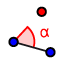How many triangles can be created?

Topic:
Triangles

You will be required to construct triangles from given information.

Part A.Draw line segment AB=10.At A, construct an angle of 30 degrees.Construct a ray from A to B'.Using B as a center, draw an circle with a radius of 6.Find a point(s) of intersection with the ray and circle. Label these C.  How many points of intersection are there? Therefore, how many different triangles ABC may be constructed?

Repeat the instructions. Each time, the radius changes.

B. Draw the circle with a radius of 5. C. Draw the circle with a radius of 3. D. Draw the circle with a radius of 12. For each part, how many triangles are possible?